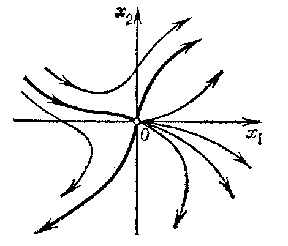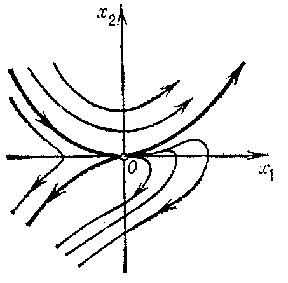##### Actions

A type of arrangement of the trajectories in a neighbourhood of a singular point $x _ {0}$ of an autonomous system of second-order ordinary differential equations

$$\tag{* } \dot{x} = f ( x),\ \ x \in \mathbf R ^ {2} ,\ \ f: G \rightarrow \mathbf R ^ {2} ,$$

$f \in C ( G)$, where $G$ is the domain of uniqueness. This type is characterized as follows. Suppose that a certain neighbourhood $U$ of $x _ {0}$ is partitioned into $m$( $3 \leq m < + \infty$) curvilinear sectors (cf. Sector in the theory of ordinary differential equations) by semi-trajectories (the separatrices of the saddle node) approaching $x _ {0}$. Suppose that $h$ of these sectors, $2 \leq h < m$, are saddle sectors and that the others are open nodal sectors, and suppose also that each semi-trajectory approaching $x _ {0}$, completed with $x _ {0}$, touches it in a definite direction. Then $x _ {0}$ is called a saddle node.

A saddle node is unstable in the sense of Lyapunov (cf. Lyapunov stability). Its Poincaré index is $1 - ( h/2)$( cf. Singular point). If $f \in C ^ {1} ( G)$ and the matrix $A = f ^ { \prime } ( x _ {0} ) \neq 0$, then the singular point $x _ {0}$ can be a saddle node for (*) only when the eigenvalues $\lambda _ {1} , \lambda _ {2}$ of $A$ satisfy one of the following conditions:

a) $\lambda _ {1} = 0 \neq \lambda _ {2}$;

b) $\lambda _ {1} = \lambda _ {2} = 0$.

In any of these cases $x _ {0}$ can also be a saddle or a node for (*), and in case b), also a point of another type. If it is a saddle node, then $m = 3$, $h = 2$, and all the semi-trajectories of the system that approach $x _ {0}$ touch at this point the directions defined by the eigenvectors of $A$( see Fig. aand Fig. b, where the heavy lines are the separatrices at the saddle node $x _ {0} = 0$, and the arrows indicate the direction of motion along the trajectories of the system as $t$ increases; they can also be in the opposite direction).Figure: s083040aFigure: s083040b

The flow near a saddle node does not enjoy structural stability: If $x _ {0}$ is a saddle node for (*), there is a neighbourhood $N$ of $x _ {0}$ in $\mathbf R ^ {2}$ such that for any $\epsilon > 0$ there is a system $\dot{x} = y( x)$ having no equilibrium in $N$, such that $| f- g | < \epsilon$ and $| ( \partial f / \partial x _ {i} ) - ( \partial g/ \partial x _ {i} ) | < \epsilon$, $i = 1, 2$. However, the saddle node bifurcation is robust and cannot be perturbed away ([a1]) (cf. also Rough system).